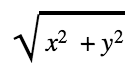# Length Node

## Description

Returns the length of input In. This is also known as magnitude. A vector's length is calculated with Pythagorean Theorum.

The length of a Vector 2 can be calculated as:Where x and y are the components of the input vector. Length can be calculated for other dimension vectors by adding or removing components.And so on.

## Ports

Name Direction Type Description
In Input Dynamic Vector Input value
Out Output Vector 1 Output value

## Generated Code Example

The following example code represents one possible outcome of this node.

``````void Unity_Length_float4(float4 In, out float Out)
{
Out = length(In);
}
``````